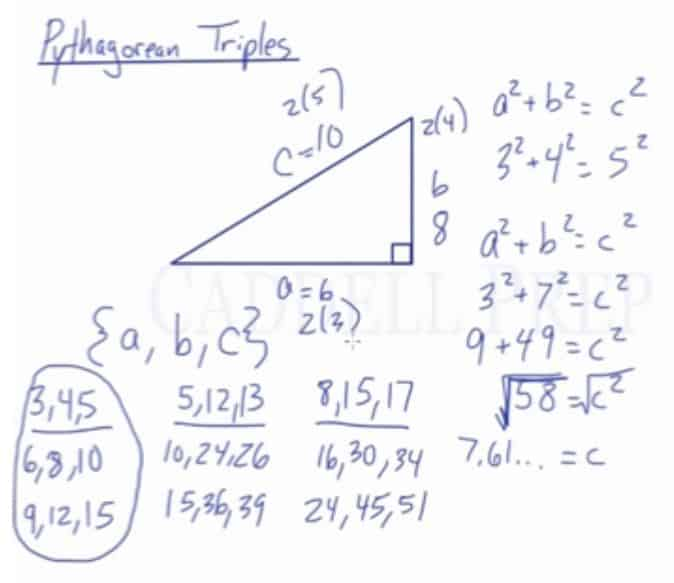In this video, we are going to look at Pythagorean Triples. From the previous video, we already know that the formula for the Pythagorean theorem is:$a^2+b^2=c^2$

Many times in mathematics, we will often end up with decimal answers when solving for a missing side by applying the Pythagorean theorem. Pythagorean triples, however, are whole numbers.

Pythagorean Triples: Series of three whole numbers that make up sides of a right triangle

Only select integers are included in Pythagorean triples:
{3, 4, 5}
{5, 12, 13}
{8, 15, 17}

If we multiply each integer by other integers, we will arrive at other triples:
{3, 4, 5}$(\times2)=$ {6, 8, 10}
{3, 4, 5}$(\times3)=$ {9, 12, 15}
{5, 12, 13}$(\times2)=$ {10, 24 26}
{5, 12, 13}$(\times3)=$ {15, 36, 39}
{8, 15, 17}$(\times2)=$ {16, 30, 34}
{8, 15, 17}$(\times3)=$ {24, 45, 51}

Knowing these will save a lot of time by eliminating the need to use Pythagorean theorem every time.Video-Lesson Transcript

Let’s go over Pythagorean triples.

We know that Pythagorean theorem is$a^2 + b^2 = c^2$

where$a$ and$a$ are the legs and$c$ is the hypotenuse

Sometimes we’re doing Pythagorean theorem and searching for one of the sides and we end up a decimal answer. Only select integers work in here.

If we know that$3^2 + 4^2 = 5^2$

But it would be different if we have different numbers.$a^2 + b^2 = c^2$$3^2 + 7^2 = c^2$$9 + 49 = c^2$$58 = c^2$$\sqrt{58} = \sqrt{c^2}$$7.6157731 = c$

Here, we did not get an integer answer.

We’ll only get an integer when we get a square root of a perfect square. Like square root of 49, square root of 64, square root of 144, all of those will give us an integer.

Pythagorean triples are a group of integers that work in this perfectly.

We’d like to list some of them:

{$a, b, c$}$3, 4, 5$

Another common one is$5, 12, 13$

Another not so common one is$8, 15, 17$

These are not the only Pythagorean triples. There’s a lot more.

We can list some of them based on these triples.

We can just multiply these by any integer and we’ll get another triple.

If we take$3, 4, 5$ multiplied by$2$, we’ll get$6, 8, 10$ which is another triple.

When multiplied them by$3$, we’ll have$9, 12, 15$.

Same thing with$5, 12, 13$.

We can double them into$10, 24, 26$ and when multiplied by$3$ we’ll get$15, 36, 39$.

We can also double$8, 15, 17$ and get$16, 30, 34$. Let’s triple it we’ll have$24, 45, 51$.

These are the most common ones we’re going to see.

The reason why we need to know these is it can save us some time writing the Pythagorean theorem.

For example we have$a = 6$ and$c = 10$, we know that this is just a$3, 4, 5$ triangle multiplied by$2$.

Here, we know that$b = 8$.

Sometimes, knowing these triples could save us from doing Pythagorean theorem.

But of course, if we know that one side is$6$ and the hypotenuse is$10$, we can find the missing side just by using Pythagorean theorem as well.